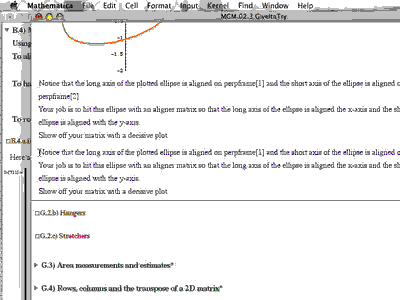# Linear Algebra

Linear Algebra is a sophomore-level introductory course to the subject.

Traditional approaches to the subject include learning tedious manual computations on matrices, followed by an introduction to a more abstract approach to looking at a class of examples called linear spaces.

Our approach in this course is not a traditional one. In the words of the authors of the curriculum, "This is not your mother's (or father's) linear algebra course", referring to the fact that someone who took an introductory linear algebra course years ago would not recognize much similarity with this course.

Leveraging the high-powered computer algebra and graphing system Mathematica™ by Wolfram Research, the course curriculum Matrices, Geometry, & Mathematica by Davis/Porta/Uhl bypasses the traditional manual calculation tedium, and leapfrogs to a computationally-based, geometric, experimentation-centered approach to the subject. Instead of learning manual computations that are today easily completed by any computer algebra system, this course races into topics that are seldom found in any linear algebra textbook - a quite unique, fresh, and powerful approach to the subject.

Students completing this Matrices, Geometry, & Mathematica curriculum will have a thorough understanding of the geometry of linear algebra, the solutions of linear systems of equations, and the theoretical investigation of the generalized linear spaces concept (although only lightly dabbling in "proofs" - just the right amount for this course level).

# Examples of the Curriculum

Below are some PDF "print outs" of a few of the Mathematica™ notebooks from Matrices, Geometry, & Mathematica by Davis/Porta/Uhl. Included as well is an example homework notebook completed by a student in the course, demonstrating how the homework notebooks become the "common blackboards" that the students and instructor both write on in their "conversation" about the notebook.

# That Looks Like Programming Code!

Yes, Mathematica™ is a syntax-based computer algebra system - i.e. the instructions to generate the graphs and computations look like a programming language code (which it is).

This course is not a course on programming. We do not teach programming, nor do we expect the students to learning programming, or even to know anything about programming. The mathematics is what is important in this course, not the code.

With that tenet in mind, the authors of the Matrices, Geometry, & Mathematica courseware have designed the explanation notebooks (Basics & Tutorials) and the homework notebooks (Give It a Try) in such a way as to make it easy to Copy/Paste from the explanations into the homework notebooks, and make minor changes (obvious ones) to produce the desired similar (but different) output. In this way, we are able to stick strickly to the mathematics at hand, and deal with the programming code as minimally as possible.# Course Catalog Listing

MAT 4110: Linear Algebra [4 credit hours]

Course Description: Topics covered include solutions of systems of linear equations, matrices, linear transformations, bases and linear independence, determinants, orthogonality, singular values, eigenvectors and eigenvalues, rank, geometric applications.

Prerequisite: Calculus II